NEET  >  Force on Current Carrying Conductor

# Force on Current Carrying Conductor Notes | Study Physics Class 12 - NEET

## Document Description: Force on Current Carrying Conductor for NEET 2022 is part of Physics Class 12 preparation. The notes and questions for Force on Current Carrying Conductor have been prepared according to the NEET exam syllabus. Information about Force on Current Carrying Conductor covers topics like and Force on Current Carrying Conductor Example, for NEET 2022 Exam. Find important definitions, questions, notes, meanings, examples, exercises and tests below for Force on Current Carrying Conductor.

Introduction of Force on Current Carrying Conductor in English is available as part of our Physics Class 12 for NEET & Force on Current Carrying Conductor in Hindi for Physics Class 12 course. Download more important topics related with notes, lectures and mock test series for NEET Exam by signing up for free. NEET: Force on Current Carrying Conductor Notes | Study Physics Class 12 - NEET
 1 Crore+ students have signed up on EduRev. Have you?

3. FIELD DUE TO A STRAIGHT CURRENT CARRYING WIRE

3.1 WHEN THE WIRE IS OF FINITE LENGTH

Consider a straight wire segment carrying a current i and there is a point P at which magnetic field to be calculated as shown in the figure. This wire segment makes angle θ1 and θ2 at that point with normal OP. Consider an element of length dy at a distance y from O and distance of this element from point P is r and line joining P to Q makes an angle q with the direction of current as shown in figure. Using Biot-Savart Law magnetic field at point P due to small current element is given by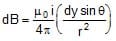As every element of the wire contributes to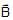in the same direction, we have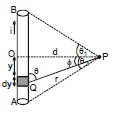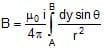....(i)

From the triangle OPQ as shown in diagram, we have

y = d tan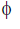or dy = d sec2dand is same triangle,

r = d secand q = (90º -), whereis angle between line OP and PQ

Now equation (i) can be written in this form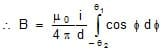or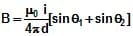...(3)

Note : θ1 & θ2 must be taken with sign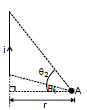For the case shown in figure

B at A =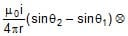Direction of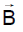The direction of magnetic field is determined by the cross product of the vector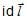with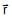. Therefore, at point P, the direction of the magnetic field due to the whole conductor will be perpendicular to the plane of paper and going into the plane.

Right-hand Thumb Rule : The direction of B at a point P due to a long, straight wire can be found by the right-hand thumb rule. The direction of magnetic field is perpendicular to the plane containing wire and perpendicular from the point. The orientation of magnetic field is given by the direction of curl fingers if we stretch thumb along the wire in the direction of current. Refer figure.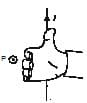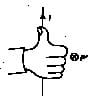Conventionally, the direction of the field perpendicular to the plane of the paper is represented by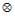if into the page and by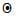if out of the page.

Now consider some special cases involving the application of equation (3)

Case 1 : When the point P is on the perpendicular bisector

In this case angle θ1 = θ2, using result of equation (3), the magnetic field is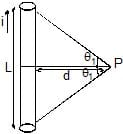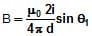where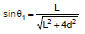Case - 2

(i) If the wire is infinitely long then the magnetic field at `P' (as shown in the figure) is given by (using q1 = q2 = 90° and the formula `B' due to straight wire)

B =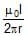⇒ B µ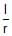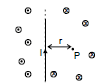The direction ofat various is as shown in the figure. The magnetic lines of force will be concentric circles around the wire (as shown earlier)

(ii) If the wire is infinitely long but `P' is as shown in the figure. The direction ofat various points is as shown in the figure. At `P'

B =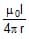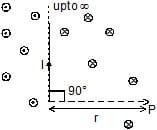Case III : When the point lies along the length of wire (but not on it)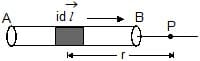If the point P is along the length of the wire (but not one it), then as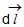and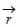will either be parallel or antiparallel, i.e., q = 0 or p, so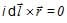and hence using equation (1)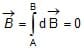Ex-1 Calculate the magnetic field induction at a point distance,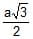metre from a straight wire of length `a' metre carrying a current of i amp. The point is on the perpendicular bisector of the wire.

Sol. B =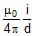[sinq1 + sinq2]

= 10-7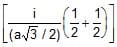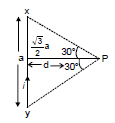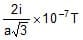Perpendicular to the plane of figure (inward).

Ex.2 Find resultant magnetic field at `C' in the figure shown.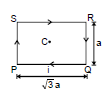Sol. It is clear that `B' at `C' due all the wires is directed Ä. Also B at `C due PQ and SR is same. Also due to QR and PS is same

Therefore, Bres = 2(BPQ + BSP)

BPQ = (sin 60° + sin 60°)

BSP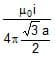(sin 30° + sin 30°)

⇒ Bres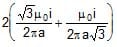=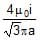Ex.3 Figure shows a square loop made from a uniform wire. Find the magnetic field at the centre of the square if a battery is connected between the points A and C.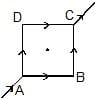Sol. The current will be equally divided at A. The fields at the centre due to the currents in the wires AB and DC will be equal in magnitude and opposite in direction. The resultant of these two fields will be zero. Similarly, the resultant of the fields due to the wires AD and BC will be zero. Hence, the net field at the centre will be zero.

Ex.4 In the figure shown there are two parallel long wires (placed in the plane of paper) are carrying currents 2 I and I consider points A, C, D on the line perpendicular to both the wires and also in the plane of the paper. The distances are mentioned.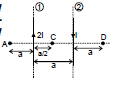Find (i)at A, C, D

(ii) position of point on line A C D whereis zero.

Sol. (i) Let us calldue to (1) and (2) as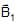and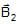respectively. Then

at A :is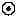andis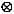B1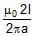and B2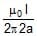Therefore, Bres = B1 - B2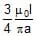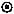Ans.

at C:isandalsoTherefore, Bres = B1 + B2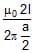+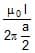=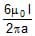=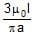Ans.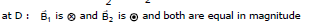Therefore, Bres = 0 Ans.

(ii) It is clear from the above solution that B = 0 at point `D'.

The document Force on Current Carrying Conductor Notes | Study Physics Class 12 - NEET is a part of the NEET Course Physics Class 12.
All you need of NEET at this link: NEET

## Physics Class 12

157 videos|425 docs|213 tests
 Use Code STAYHOME200 and get INR 200 additional OFF

## Physics Class 12

157 videos|425 docs|213 tests

### How to Prepare for NEET

Read our guide to prepare for NEET which is created by Toppers & the best Teachers

Track your progress, build streaks, highlight & save important lessons and more!

,

,

,

,

,

,

,

,

,

,

,

,

,

,

,

,

,

,

,

,

,

;